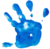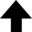Science appears to us with a very different aspect after we have found out that it is not in lecture rooms only … but that we may find illustrations of the highest doctrines of science in games and gymnastics, in travelling by land and by water, in storms of the air and of the sea, and wherever there is matter in motion.James Clerk Maxwell

This program has an interesting history. Many moons ago, I obtained it from my thesis advisor—at the time it was written in C by B.A. Weaver. (It's currently maintained on GitHub.) It was somewhat antiquated; for example, it could not manipulate complex numbers natively. I then translated it into the more appropriate FORTRAN 77, cleaning up the code and removing some logic problems. More recently I've been on a kick of modernizing older Fortran programs to the current standard, and so the program (which I call Dreamweaver for historical reasons) was washed through that process as well.

By way of introduction, the first utility one encounters in the program is tasked with creating and setting to 0 all entries in the external absorber and range tables. It also sets up the energy table by creating a logarithmically uniform distribution of energies between a minimum and a maximum energy, with the number of entries given by MAXE. The routine dEdx is the $\mathrm{d} E/\mathrm{d} x$ calculator, and is the core of the whole package. Values of certain physical constants as well as some corrections to the basic stopping power formulae have been updated. For more details the interested reader should consult the sources

## Description of the CalculatorThis program provides range, initial kinetic energy, final kinetic energy, or linear energy transfer for a specified projectile-target combination. The interface is task-driven, which the user can select from the drop-down menu below.

Range — Please see Notes for the Range-Energy Calculator for details on how the program calculates this. Returns range in g/cm2.

Initial Kinetic Energy — This task obtains the initial kinetic energy of the projectile by first finding the placement of depth within the range table trange(abs,i), then interpolating these neighboring values in terms of the energy table tenerg(i). Returns initial kinetic energy in MeV/n.

Final Kinetic Energy — This task integrates $\mathrm{d} E/\mathrm{d} x$ to find the change in kinetic energy, with the initial kinetic energy pointed to by second (in MeV/n), and the target thickness (depth) pointed to by third (in g/cm2). Returns final kinetic energy in MeV/n.

Linear Energy Transfer — This task is a straight evaluation of $\mathrm{d} E/\mathrm{d} x$, including any stopping power corrections the user chooses. Here second has the same meaning as in the $\text{KE}_f$ task, but third now acts as a switch for Restricted Energy Loss (REL). Returns LET in keV/$\mu$m.

You can download a write-up of stopping power theory here [PDF].

### Stopping Power CorrectionsThese are the stopping power corrections available. The defaults, marked in red, are appropriate for most situations.

FD — The density effect correction $\delta/2$ accounts for the phenomenon that, in a dense medium, the field which perturbs electrons far from the projectile track is modified by the dielectric polarization of the atoms between the distant electrons and the projectile.

FE — The correction due to electron capture accounts for the fact that the bare nuclear charge of the projectile $Z_0$ is reduced and can thus be replaced by an effective projectile charge $Z_1$. FE is the only correction needed for "slow" alpha particles emitted during, e.g., natural radioactivity.

FSH — The shell correction arises when the velocity of the projectile is comparable to the velocities of the electrons in the target atoms. Why? The Bethe-Bloch stopping power formula is derived on the assumption that the velocity of the incident particle is large compared to the velocities of the atomic electrons with which it interacts. When this condition is not satisfied, shell corrections are needed which are available for heavy particles but not for electrons.

FLE — The Leung, or relativistic shell correction, is a small effect which is due to relativistic inner shell electrons in very heavy targets. See P.T. Leung, Phys. Rev. A 40, 5417 (1989) and P.T. Leung, Phys. Rev. A 60, 2562 (1999) for details.

FKIN — The kinematic correction $f_\text{FKIN}$ accounts for the finite mass of the nucleus in electron-projectile collisions. This is an estimate of the ultrarelativistic kinematic correction from S.P. Ahlen, Rev. Mod. Phys. 52, 121 (1980). It is a correction to the finite mass (as opposed to size) of the nucleus in relativistic electron-nucleus collisions.

FRAD — This is the radiative correction $f_\text{FRAD}$ discussed in S.P. Ahlen, Rev. Mod. Phys. 52, 121 (1980). It arises from bremsstrahlung of scattered electrons in ultrarelativistic collisions. The form here is that of V.Z. Jankus, Phys. Rev. 90, 4 (1953). The parameter $Q$ from that paper is here equal to the geometric mean between the electron rest energy and $2 m_\text{e} c^2 \gamma$.

FLS — The Lindhard-Sørensen (LS) correction $f_\text{FLS}$ replaces the Bloch-Mott-Ahlen group. In their paper Lindhard and Sørensen show that the low energy limit of the LS correction is exactly the Bloch correction. Furthermore, by using exact solutions to the Dirac equation, the LS correction automatically incorporates Mott scattering and is relativistically correct.

FNS — In their paper Lindhard and Sørensen also derive a correction due to the finite size of atomic nuclei. This is possible because exact solutions to the Dirac equation exist for any spherically symmetric potential.

FBA — The factor $f_\text{FBA}$ takes into account target polarization effects for low energy distant electronic collisions that produce a multiplicative correction to the energy loss.

FBR — This correction $f_\text{FBR}$ accounts for bremsstrahlung emitted directly from the projectile in the effective field of the target nuclei.

### A Very Short Introduction to the Bethe-Bloch FormulaThe interaction of heavy charged particles with the electrons in a stopping medium (absorber) is described by the Bethe-Bloch formula. (The quantity $\mathrm{d} E/\mathrm{d} x$ is sometimes preceded by a negative sign to indicate that kinetic energy is transferred to the stopping medium.) A charged particle or ion projectile with atomic number $Z$ of rest mass significantly larger than that of the electron (charge $e$) is considered a "heavy" charged particle. This would include mesons, protons, alpha particles, and higher $Z$ nuclei. Electrons and positrons are considered "light" charged particles (see more at Stopping Power of Electrons).

The quantities in the Bethe-Bloch formula (below) have the following meanings: $e^2 / 4 \pi \epsilon_0$ is the coupling strength of electromagnetism; $m_\text{e} c^2$ is the rest mass-energy of the electron; $\rho \bar{Z} N_\text{A} / \bar{A}$ is the electron density of the absorber with effective atomic number $\bar{Z}$ and effective atomic mass $\bar{A}$ (when referring to compounds and mixtures).

### References• "PSTAR: Stopping Power and Range Tables for Protons." National Institute of Standards and Technology, Physical Measurement Laboratory.
• "ASTAR: Stopping Power and Range Tables for Helium Ions." National Institute of Standards and Technology, Physical Measurement Laboratory.

## Interaction of Ionizing Radiation with Matter

This program provides range, initial kinetic energy, final kinetic energy, or linear energy transfer for a specified projectile-target combination. Please be patient—these calculations can be lengthy.
$\displaystyle{ - \left \langle \frac{\mathrm{d} E}{\mathrm{d} x} \right \rangle = \underbrace{ \left( \frac{e^2}{4 \pi \epsilon_0} \right)^2 }_\text{Coulomb} \underbrace{ \frac{4 \pi Z^2}{m_\mathrm{e} c^2 \beta^2} }_\text{projectile} \underbrace{ \frac{\rho \bar{Z} N_\text{A}}{\bar{A}} }_\text{target} \left[ \ln{ \left( \frac{2 m_\mathrm{e} c^2 \beta^2}{I (1 - \beta^2)} \right) } f_\text{FBA} - \beta^2 - \frac{\delta}{2} + f_\text{FLS} + f_\text{FKIN} + f_\text{FRAD} \right] + f_\text{FBR} \text{,} }$

where $\beta \equiv v /c = \sqrt{1 - \left[ m_\text{e} c^2 / (E + m_\text{e} c^2) \right]^2 }$ from special relativity, and $I$ is the mean ionization potential.

Projectile
MeV/n
g/cm2
Target
g/cm2
Corrections
 FD FE FSH FLE FKIN FRAD FLS FNS FBA FBR
Result

( cm) in

0%